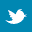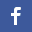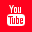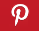Completely Solved C, C++ Programs Assignment.Quick Search Database Videos Tutorials Ebooks Forums FAQ Aboutus Household Industrial Manufacturing Service Shopping Transportation### C program to add all the elements of a 2D matrix row-wise, and display the elements of the row with the highest sum.

 Filed Under: C Assignments

PROBLEM STATEMENT:
Write a C program to add all the elements of a 2D matrix row-wise, and display the elements of the row with the highest sum.

THEORY:
In the 2D array the elements are stored in row-major form. To traverse the array, row-major wise, the variable pointing to the row is kept constant and the variable pointing to the column is incremented. In this way the rows are traversed one by one and the sum of the elements of a row is stored at the last location of the row. The variable pointing to the row is incremented and the one pointing to the column is re-initialized with 0, to start of operations with a new row. Meanwhile, a variable in maintained which is updated with the highest sum as soon as it is encountered. Lastly, this variable is matched with the last column of the array, and the particular row is printed whose sum of elements matches the content of this variable.

ALGORITHM:
Begin
Begin function main
Read r1 //Row size of Array 1
Read c1 //Column size of Array 1
Read r2 //Row size of Array 2
Read c2 //Column size of Array 2

Allocate r1 and c1 to array a[ ][ ] and r2 and c2 to array b[ ][ ]

Print ’Enter elements of Matrix 1’
for iß0 to less than r1, increment i by 1
for jß0 to less than c1, increment j by 1

Print ‘Enter elements of Matrix 2’
for iß0 to less than r2, increment i by 1
for jß0 to less than c2, increment j by 1

do
switch(ch)//Switching through the different functions according to choice
break
case 2:sub(a,b,r1,c1,r2,c2)
break
case 3:mul(a,b,r1,c1,r2,c2)
break
case 4:Print ‘Terminating program’
break
if ch is not equal to any of the mentioned cases
Print ‘Wrong choice entered’
While ch is not equal to 4
End of function main

Begin function add(int **a,int **b,int r1,int c1,int r2,int c2)
if r1 is equal to r2 and c1 is equal to c2)
Print ‘The summation matrix is’
for iß0 to less than r1,increment i by 1
for jß0 to less than c1, increment j by 1
tempßa[i][j]+b[i][j]
Print temp
New Line
else
Print ‘The matrices cannot be added as we have different row and column no.s for the two matrices’

Begin function sub(int **a,int **b,int r1,int c1,int r2,int c2)
if r1 is equal to r2 and c1 is equal to c2)
Print ‘The difference matrix is’
for iß0 to less than r1,increment i by 1
for jß0 to less than c1, increment j by 1
tempabsolute value of (a[i][j]-b[i][j])
Print temp
New Line
else
Print ‘The difference cannot be found out as we have different row and column no.s for the two matrices’
End function sub

Begin function mul(int **a,int **b,int r1,int c1,int r2,int c2)
if c1 is equal to r2 //if row of 1st array and column of 2nd array is equal
for iß0 to less than r1,increment I by 1
for jß0 to less than c2, increment j by 1
sumß0
for kß0 to less than r2, increment k by 1 //loop to generate column of 1st and row of 2nd array
sumßsum+(a[i][k]*b[k][j])
Print sum
Newline
else
Print ’The matrices could not be multiplied because no. of columns in first matrix is not equal to no. of rows in second matrix’
End of function mul
End

PROGRAM LISTING:
//Program to print the row with the highest sum
#include<stdio.h>
#include<conio.h>
#include<malloc.h>

void main()
{
int **a,r,c,i,j,ans,max;
clrscr();
//Accepting size of row and column
printf("Enter size of row n");
scanf("%d",&r);
printf("Enter size of column n");
scanf("%d",&c);

//Allocating memory to array
a=(int **)malloc(r*sizeof(int *));
for(i=0;i<r;i++)
a[i]=(int *)malloc((c+1)*sizeof(int));

printf("Enter array elements n");

for(i=0;i<r;i++)
for(j=0;j<c;j++)
scanf("%d",&a[i][j]); //Accepting array elements from user

printf("The array is : n");

for(i=0;i<r;i++)
{
for(j=0;j<c;j++)
printf("%d ",a[i][j]); //Printing inputted array
printf("n");
}

for(i=0;i<r;i++)
{
ans=0;
for(j=0;j<c;j++)
{
ans=ans+a[i][j];//Sum of 'i'th row
if(i==0)
max=ans;//initializing max for the very first iteration
if(ans>=max)

max=ans;
a[i][c]=max;
}//end of if
}//end of loop j
}//end of loop i

//Printing
printf("The row with the highest sum is : n");
for(i=0;i<r;i++)
{
if(a[i][c]==max) //Check for multiple rows with similar maximum values
{
for(j=0;j<c;j++)
printf(" %d ",a[i][j]);
printf("n");
}//end of if
}//end of loop i
}//end of main

OUTPUT:
Enter size of row
6
Enter size of column
6
Enter array elements
1
2
3
4
5
6
7
8
9
10
11
12
13
14
15
16
17
18
17
16
15
14
13
12
11
10
11
9
8
7
6
5
4
3
2
1
The array is :
1 2 3 4 5 6
7 8 9 10 11 12
13 14 15 16 17 18
17 16 15 14 13 12
11 10 11 9 8 7
6 5 4 3 2 1
The row with the highest sum is :
13 14 15 16 17 18

DISCUSSION:
The variable which stores the highest sum of rows is not initialized with 0 or with any arbitrary number but with the sum of the elements of the first row to prevent any error arising if the sum of elements of a particular row happens to be 0 or less than the value with which the variable has been initialized.
The sum of each row is stored in the last cell of a particular row so that it can be used for comparison later. This value is compared with the value of the variable storing the highest sum right at the end and the elements of the row are printed if there is a match. This is helpful if there are multiple rows with the same sum of their elements.Back to main directory:Software Practical